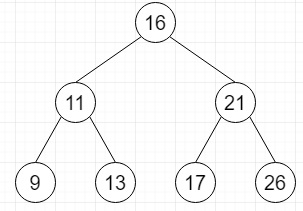# Find a pair with given sum in a Balanced BST in C++

Suppose we have a balanced binary search tree and a target sum, we have to define a method that checks whether it is a pair with sum equals to target sum, or not. In this case. We have to keep in mind that the Binary Search Tree is immutable.

So, if the input is likethen the output will be (9 + 26 = 35)

To solve this, we will follow these steps −

• Define stacks s1, s2
• done1 := false, done2 := false
• val1 := 0, val2 := 0
• curr1 := root, curr2 := root
• infinite loop, do −
• while done1 is false, do −
• if curr1 is not equal to NULL, then &minus
• insert curr1 into s1
• curr1 := left of curr1
• Otherwise
• if s1 is empty, then −
• done1 := 1
• Otherwise
• curr1 := top element of s1
• delete element from s1
• val1 := val of curr1
• curr1 := right of curr1
• done1 := 1
• while done2 is false, do −
• if curr2 is not equal to NULL, then −
• insert curr2 into s2
• curr2 := right of curr2
• Otherwise
• if s2 is empty, then −
• done2 := 1
• Otherwise
• curr2 := top element of s2
• delete element from s2
• val2 := val of curr2
• curr2 := left of curr2
• done2 := 1
• if val1 is not equal to val2 and (val1 + val2) is same as target, then −
• print pair (val1 + val2 = target)
• return true
• otherwise when (val1 + val2) < target, then −
• done1 := false
• otherwise when (val1 + val2) > target, then −
• done2 := false
• if val1 >= val2, then −
• return false

## Example

Let us see the following implementation to get better understanding −

Live Demo

#include <bits/stdc++.h>
using namespace std;
#define MAX_SIZE 100
class TreeNode {
public:
int val;
TreeNode *left, *right;
TreeNode(int data) {
val = data;
left = NULL;
right = NULL;
}
};
bool isPairPresent(TreeNode* root, int target) {
stack<TreeNode*> s1, s2;
bool done1 = false, done2 = false;
int val1 = 0, val2 = 0;
TreeNode *curr1 = root, *curr2 = root;
while (true) {
while (done1 == false) {
if (curr1 != NULL) {
s1.push(curr1);
curr1 = curr1->left;
}
else {
if (s1.empty())
done1 = 1;
else {
curr1 = s1.top();
s1.pop();
val1 = curr1->val;
curr1 = curr1->right;
done1 = 1;
}
}
}
while (done2 == false) {
if (curr2 != NULL) {
s2.push(curr2);
curr2 = curr2->right;
}
else {
if (s2.empty())
done2 = 1;
else {
curr2 = s2.top();
s2.pop();
val2 = curr2->val;
curr2 = curr2->left;
done2 = 1;
}
}
}
if ((val1 != val2) && (val1 + val2) == target) {
cout << "Pair Found: " << val1 << " + " << val2 << " = " << target << endl;
return true;
}
else if ((val1 + val2) < target)
done1 = false;
else if ((val1 + val2) > target)
done2 = false;
if (val1 >= val2)
return false;
}
}
int main() {
TreeNode* root = new TreeNode(16);
root->left = new TreeNode(11);
root->right = new TreeNode(21);
root->left->left = new TreeNode(9);
root->left->right = new TreeNode(13);
root->right->left = new TreeNode(17);
root->right->right = new TreeNode(26);
int target = 35;
cout << (isPairPresent(root, target));
}

## Input

TreeNode* root = new TreeNode(16);
root->left = new TreeNode(11);
root->right = new TreeNode(21);
root->left->left = new TreeNode(9);
root->left->right = new TreeNode(13);
root->right->left = new TreeNode(17);
root->right->right = new TreeNode(26);

## Output

Pair Found: 9 + 26 = 35
1